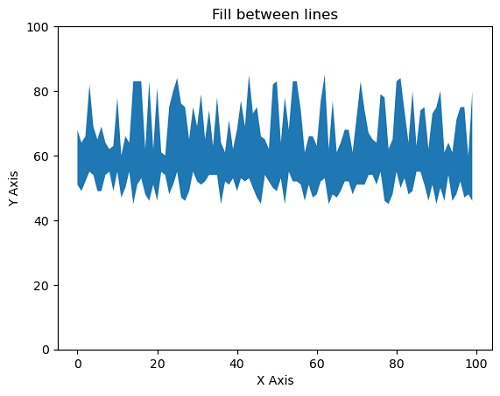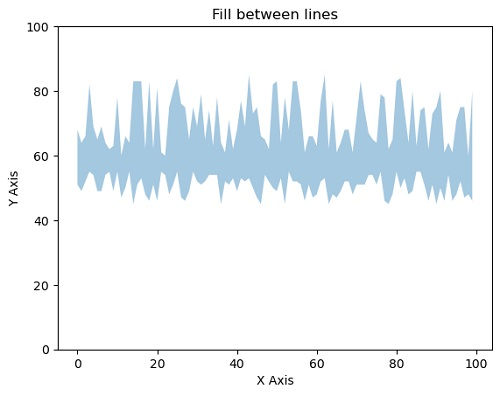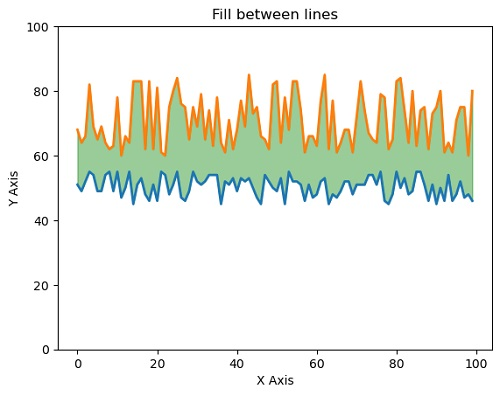# Python | Filling the area between two lines in plot using matplotlib

In this tutorial, we are going to learn how to fill the area between the lines in python using matplotlib?
Submitted by Anuj Singh, on July 24, 2020

In some of the applications, we need to fill the area covered between two lines and therefore, matplotlib has an inbuilt defined function for our desired operation i.e. matplotlib.pyplot.fill_between(). Following are the examples showing the implementation of matplotlib.pyplot.fill_between().

Illustration:

```matplotlib.pyplot.fill_between(x, y, yy)
#Filling the area between two lines completely
``````matplotlib.pyplot.fill_between(x, y, yy, alpha=0.4)
#Filling the area between two lines completely with opaque ratio 0.4
``````matplotlib.pyplot.plot(x,y, yy, linewidth=2.0)
matplotlib.pyplot.fill_between(x, y, yy, color='green', alpha=0.4)
#Filling the area between two lines completely
#in addition to show the lines as well.
```## Python code for filling the area between two lines in plot using matplotlib

```import matplotlib.pyplot as plt
import numpy as np

#random data generation
x = np.arange(100)
y = np.random.randint(45,56,100)
yy = np.random.randint(60,86,100)

#Illustration 1
plt.fill_between(x, y, yy)
plt.xlabel('X Axis')
plt.ylabel('Y Axis')
plt.ylim(0,100)
plt.title('Fill between lines')
plt.show()

#Illustration 2
plt.fill_between(x, y, yy, alpha=0.4)
plt.xlabel('X Axis')
plt.ylabel('Y Axis')
plt.ylim(0,100)
plt.title('Fill between lines')
plt.show()

#Illustration 3
plt.plot(x,y, yy, linewidth=2.0)
plt.fill_between(x, y, yy, color='green', alpha=0.4)
plt.xlabel('X Axis')
plt.ylabel('Y Axis')
plt.ylim(0,100)
plt.title('Fill between lines')
plt.show()
```

Output:

```Output is as figure
```